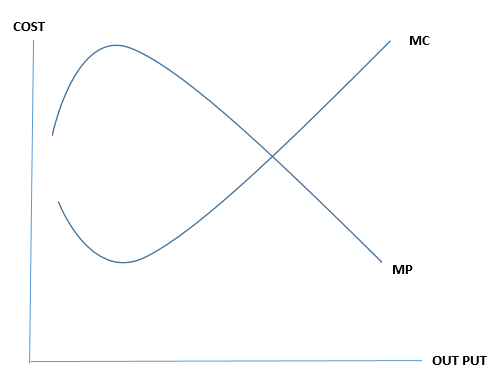# What is Diminishing Marginal Returns, Why Does It Occur?

## What is Diminishing Marginal Returns

Diminishing marginal returns is a theory in economics that states if more and more units of a variable input are applied when other inputs are held constant, the returns from the variable input may decrease eventually even though there is an initial increase. This is also known as principle of diminishing marginal productivity or diminishing returns. If it is applied to companies that have fixed stock of capital, or tools and machines, and a variable supply of labor, the total output of the firm grows at an ever-decreasing rate as the firm increases the number of workers.

### Law of Diminishing Marginal Returns

If hiring an additional factor of production causes a relatively smaller increase in output at a certain point, it  is called as the law of diminishing marginal return.

## Why Do Diminishing Marginal Returns Occur

If the variable factor of production increases, the output will increase up to a certain point. After a certain point, that factor becomes less productive; therefore, there will eventually be a decreasing marginal return and average product. The reason behind this theorem indicates that if the capital is fixed, extra workers will eventually get in each other’s way as they attempt to increase production.

Diminishing returns occurs only in the short run when one factor is fixed; in the long run, all factors are considered as variable.

Diminishing marginal return can be explained by a practical example as follows.

 No. of labors Marginal P Total P Labor Cost Marginal C Average Cost 1 2 2 20 10 10 2 4 6 40 5 6.66 3 6 12 60 3.33 5 4 8 20 80 2.5 4 5 10 30 100 2 3.33 6 8 38 120 2.5 3.15 7 5 43 140 4 3.25 8 3 46 160 6.66 3.5

Here labor cost is considered as \$20 per labor. Marginal product (MP) is the units that can be produced by introducing an additional unit of labor. The marginal cost (MC) of a product is the ratio of cost of a worker and extra quantity that he produces. Total Product (TP) is the total output produced by workers.

In above example, 1st worker produces 2 products. The 2nd worker produces 4 products. So up to the 5th labor unit marginal product is gradually increasing. After 5th labor marginal production has started to decrease. 6th labor unit introduced produces just 8 products. From 6th labor marginal cost has increased. This can be graphically illustrated as follows.Using fertilizer in the agriculture industry is another clear example that carries the same results. Plants need an exact quantity for the more yield. Very Small quantity leads to a less output, but large quantities can also lead to a less output. Accordingly, with the increase of input, output increases at an increasing rate, then decreases, maximizes and starts to reduce. The point yield gets the highest value at the point where MP gets its maximum and MC gets its minimum value.

### Conclusion

Diminishing marginal returns is an important economic theory that explains the behavior of inputs and outputs pertaining to the production process. In general context, we believe that with the increase of the number of inputs, the number of output will increase. But diminishing marginal returns concept describes a different behavior. Accordingly, if the production process allocates more and more variable inputs, the output will increase up to a certain point before it starts to fall eventually.